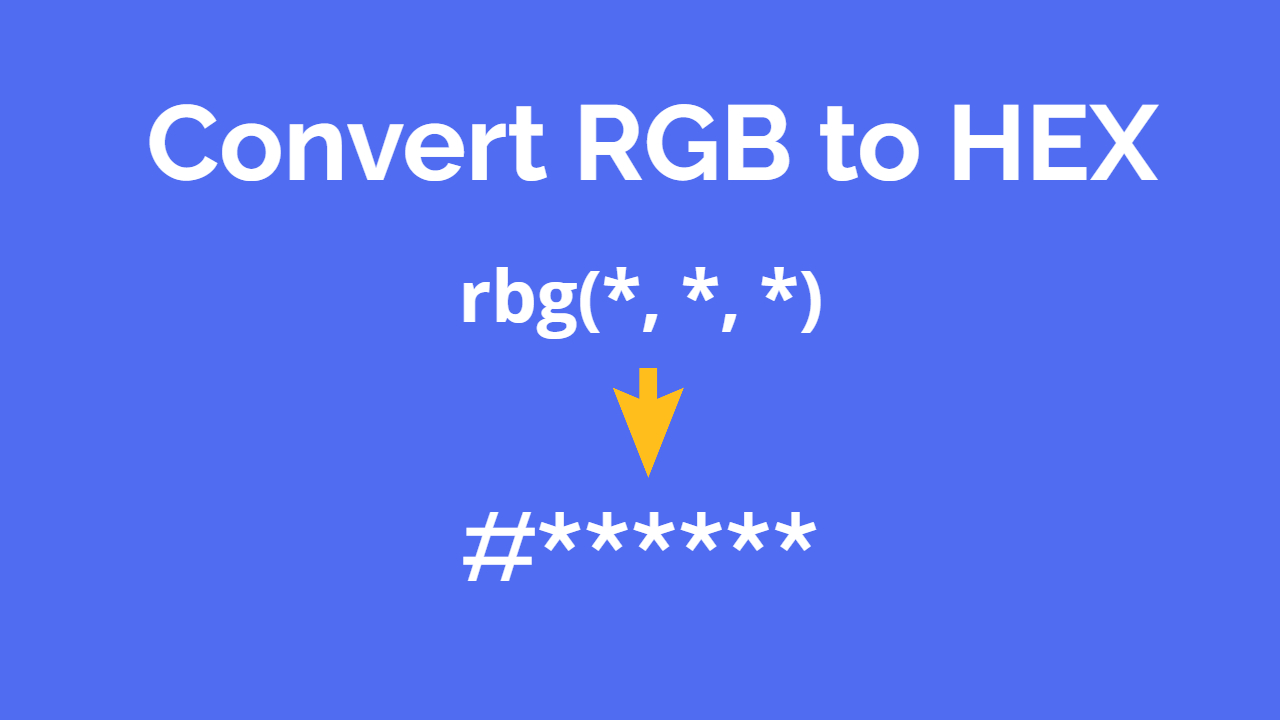Convert RGB to HEX ( Javascript Function )# Convert RGB to HEX ( Javascript Function )

Here this is simple javascript function for you that will help you to convert RGB color code to HEX try this out.
``````//Function to convert hex format to a rgb color
function rgb2hex(rgb) {
var hexDigits = ["0","1","2","3","4","5","6","7","8","9","a","b","c","d","e","f"];
rgb = rgb.match(/^rgb\((\d+),\s*(\d+),\s*(\d+)\)\$/);
function hex(x) {
return isNaN(x) ? "00" : hexDigits[(x - x % 16) / 16] + hexDigits[x % 16];
}
return "#" + hex(rgb) + hex(rgb) + hex(rgb);
}``````

you can use this function as:

``rgb2hex("rgb(244, 244, 244)");``

Thats it use it and have fun.
Disqus Codes

#### Notifications